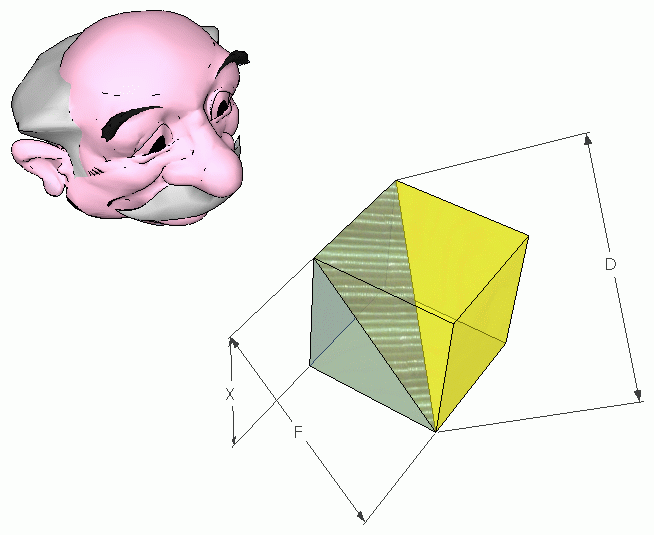SEARCH HOMEMath Central Quandaries & QueriesQuestion from Praveen, a parent: Hi, Given a Cube's diagonal, how does one find the edge of the cube. Thanks for quick response regards PraveenHi Praveen.

Let X = the length of the edge of the cube.
Let F = the length of the diagonal on a face of the cube.
Let D = the length of the diagonal through the cube (what you are given).Then use the Pythagorean Theorem:
D2 = X2 + F2

F2 = X2 + X2

So

D2 = X2 + (X2 + X2).

Finish by solving for X.

Cheers,
Stephen La Rocque.Math Central is supported by the University of Regina and The Pacific Institute for the Mathematical Sciences.